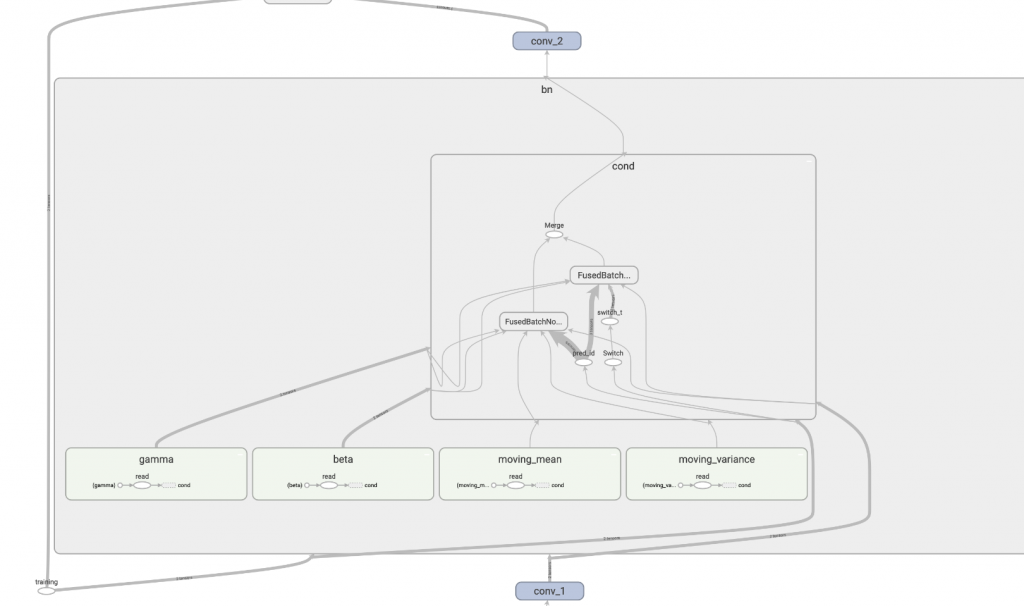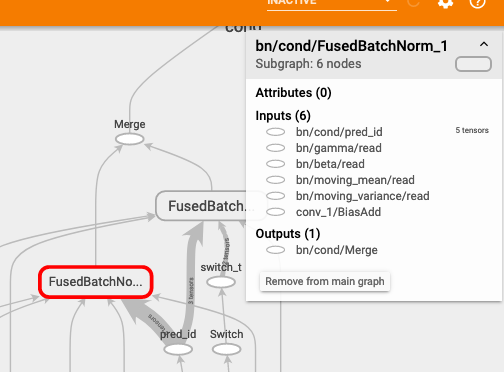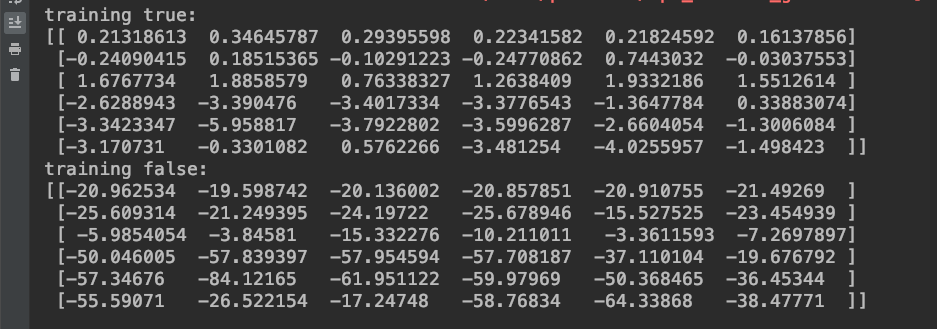DAY 10
0
Google Developers Machine Learning

【10】從 tensorboard 來觀察：你容易忽略的 batch_normalization 原理篇

(mu, sigma) 分別代表這次 batch data 的平均和標準差。
(gamma, beta) 代表要被 gradient 訓練的權重值
(running_mean, running_var) 訓練時，由觀察 batch data 得到的平均和標準差。

x = (x - mu) / sigma

out = gamma * x + beta

running_mean = momentum * running_mean + (1 - momentum) * sample_mean
running_var = momentum * running_var + (1 - momentum) * sample_var

x = (x - running_mean) / running_var

normalization 完後再一樣乘上 gamma 並加上 beta 後輸出。

out = gamma * x + beta

graph = tf.get_default_graph()

graph_def = graph.as_graph_def()
with gfile.FastGFile(MODEL_PB, 'rb') as f:
tf.import_graph_def(graph_def, name='')FusedBatchNormFusedBatchNorm_1.
.
.
.
.
.
.
.

training 使用 FusedBatchNorm，inference 使用 FusedBatchNorm_1。

input_node = graph.get_tensor_by_name(
"input_node:0")
training_node = graph.get_tensor_by_name(
"training:0")

debug_node = graph.get_tensor_by_name(
"bn/cond/Merge:0")

with tf.Session() as sess:
image = np.expand_dims(image, 0)

# 訓練
result = sess.run(debug_node, feed_dict={input_node: image, training_node: True})
print(f'training true:\n{result[0, 22:28, 22:28, 0]}')

# 推斷
result = sess.run(debug_node, feed_dict={input_node: image, training_node: False})
print(f'training false:\n{result[0, 22:28, 22:28, 0]}')github原始碼